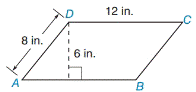Chapter 8.1, Problem 13EElementary Geometry For College St...

7th Edition
Alexander + 2 others
ISBN: 9781337614085

Solutions

Chapter
SectionElementary Geometry For College St...

7th Edition
Alexander + 2 others
ISBN: 9781337614085
Textbook Problem

In Exercises 9 to 18, find the areas of the figures shown or described.▱ A B C D

To determine

To find:

The area of ABCD.

Explanation

1) Formula:

Area of the parallelogram is given by,

A=bh unit2.

Where b is the base of the length, h is the height.

2) Calculation:

Given,

In the Parallelogram ABCD with base (b)=12 in.

Side (s)=8 in.

Height (h)=6 in

Still sussing out bartleby?

Check out a sample textbook solution.

See a sample solution

The Solution to Your Study Problems

Bartleby provides explanations to thousands of textbook problems written by our experts, many with advanced degrees!

Get Started

Find more solutions based on key concepts